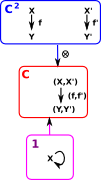# Maths - Cartesian Closed Categories

These pages discuss, not just cartesian closed categories, but also a whole hierarchy of categories.

 This diagram of the relationship between various categories is adapted from this John Baez paper.### Introduction

Category diagrams can define mathematical structures, such as monoids, from the outside. Category diagrams can also define operations on those structures, such as products, which can build structures by combining existing structures. Note that the categorical product does not necessarily define the multiplication in a concrete structure like a monoid. However there is a structure, like a monoid, but whose objects are categories, this is a monoidal category.

### Monoidal Category

We can catogorify the idea of a monoid by changing the equalities to isomorphisms.

Monoid Monoidal Category

a binary operation *

axioms:

• Associatively: (a * b) * c = a * (b * c)
• Identity element: e * a = a = a * e

a tensor product functor:C×C->C

axioms:

• Associator: (ab)ca(bc)
• unitor: 1aaa1

Pentagon### Cartesian Product

We can extend the concept of cartesian product of sets to categories as follows:

 An object of A×B is a pair (X,X'). A morphism of A×B is a pair (f,f').Composition is done componentwise: (g,g')(f,f')=(gf,gf')Identity morphisms are defined componentwise: 1(X,X') = (1X,1X')

The arrows, between A,B,A×B and Q, on the above diagram are functors, that is, they are functions between categories that preserve some structure.

The cartesian product is not necessarily commutative or associative, although it is associative upto isomorphism.

#### Example - Sets

{a,{b,c}} is not equal to {{a,b},c} but it is isomorphic to it.

### Tensor Product

The tensor product involves the following concepts:

• Combining arrows in parallel.
• Binary functor:C×C->C.
• The most general bilinear operation.
• Carteasion product.
• Outer product.
• Co-products of frames (locales).

A tensor product (i.e. a monoidal product) is not, in general, a categorical pruduct (such as a catesian product). However, the converse is, that is a cartesian product is a monoidal product.

 The tensor of A and B exists if there is an object ABC together with isomorphisms: C(AB,X)[A,C(B,X)]#### Bilinear Aspect of Tensor Product### Cartesian Category

We have seen the monoidal product (tensor product) is not necessarily the same as the categorical product (carteasian product). In cases where they are the same the monoidal category is known as a cartesian category.

Examples (from this video) of cartesian categories are:

Cartesian Category Group Object
Set group
Top topological group
Manifold Lie group
Group abelian group
Cat crossed module

A category has finite products if it has a binary product and a terminal object.

• The binary product is the tensor product.
• The terminal object is the unit object. The unit '1' is like a product with zero parameters.

### Braided Category

The string diagrams for a braided category can have a crossover.

## Generalisation